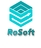### Choice field as a switch question.

I have multiple options available in a multiple choice/switch field and am wondering if it's possible to attach a value to each choice and have these values added all together in another field. I have used a if , then formula in a choice field before but not multiple values into one field and adding multiple values.  Example, extra length is one option and lt has a value of 25. Then another choice is made , extra width and it has a value of 55. I then have a seperate field named Extras and the two values from my selection will be totaled. I might have 2,3,4,5 extas . What type of field should the totals be, fx 🤷🏼‍♂️

hope this makes sense. Thank youlet c := 0;

let mc := concat('Multipe Choice');

if contains(mc,"Extra Length") then c := c + 25 else

if contains(mc,"Extra width") then c := c + 55 else

if contains(mc,"Option 3") then c := c+ 33

...

end;

c

I think this would do the trick. It might need some tweaking because this is on my phone.

StevenIt should be :

let mc := text('Multiple Choice');

Hi Steven, hope you had a nice Christmas and thank you for helping me.  I have tried this formula in a fx field and it is not working. Can you see any issues.

'Lined_Per_Width' is the Mutiple choice field. The fx Field is called 'All_Extras' set to currency.    When I make a selection in the choices the field the currency disappears and field is blank.

Michael

let c := 0;
let mc := text('Lined_Per_Width');
if contains(mc, "Interlining") then
c := c + 25
else
if contains(mc, "Curtains over 2.7") then
c := c + 55
else
if contains(mc, "Continuous Sheer Drop Over 2.7") then
c := c + 30
else
if contains(mc, "Lined Curtain Double Fold Side Hem") then
c := c + 20
else
if contains(mc, "Curtains over 5 Widths") then
c := c + 15
else
if contains(mc, "Sheers over 12.m") then
c := c + 20
else
if contains(mc, "Continuous Linings or Sheers need a join") then
c := c + 25
end;
c
end
end
end
end
end
endMichael,

This works:

`let c := 0;let mc := text(Lined_Per_Width);if contains(mc, "Interlining") then c := c + 25end;if contains(mc, "Curtains over 2.7") then c := c + 55end;if contains(mc, "Continuous Sheer Drop Over 2.7") then c := c + 30end;if contains(mc, "Lined Curtain Double Fold Side Hem") then c := c + 20end;if contains(mc, "Curtains over 5 Widths") then c := c + 15end;if contains(mc, "Sheers over 12.m") then c := c + 20end;if contains(mc, "Continuous Linings or Sheers need a join") then c := c + 25end;c`

Steven

Hi Steven , yet again you come up with the goods   Five Stars........ I am truly gateful for your assistance, I am sure I will be calling upon your brain power again in the near furure. I hope you get some sort of recognition from Ninox for your excellent advise, a very big thank you. Michael

For the sake of diversity, another way to compute it in "functional" style, illustrating the use of the "chosen" function with a second argument:

`25 * number(chosen(Lined_Per_Width, "Interlining")) + 55 * number(chosen(Lined_Per_Width, "Curtains over 2.7")) + 30 * number(chosen(Lined_Per_Width, "Continuous Sheer Drop Over 2.7")) + 20 * number(chosen(Lined_Per_Width, "Lined Curtain Double Fold Side Hem")) + 15 * number(chosen(Lined_Per_Width, "Curtains over 5 Widths")) + 20 * number(chosen(Lined_Per_Width, "Sheers over 12.m")) + 25 * number(chosen(Lined_Per_Width, "Continuous Linings or Sheers need a join"))`Alain,

At first glance I thought this wouldn work but yeah, it also works your way.

chosen(Lined_Per_Width,"Interlining") gives Yes if the button is active and No if it's not.

Then with number() you make this a 1 or a 0 so 25 * 0 gives 0 to count up.

Thanks for pointing that up. (Wondering which code would run faster for the sake of speed, I guess your code is faster)

Also curious which code you would use yourself to keep the overview in case debugging is needed ...

It is difficult to make an assumption on the performance aspect, not knowing what kind of optimization or maybe pre-compilation Ninox does internally. Something that would probably speed things up could be to use the form of "chosen()" where the reference number of the choices is given instead of the text form. This has the added advantage that, if the text of some option must be modified, the formula does not need to be corrected.

25 * number(chosen(Lined_Per_Width, 1)) + 55 * number(chosen(Lined_Per_Width, 2)) + 30 * number(chosen(Lined_Per_Width, 3)) + 20 * number(chosen(Lined_Per_Width, 7)) + 15 * number(chosen(Lined_Per_Width, 4)) + 20 * number(chosen(Lined_Per_Width, 5)) + 25 * number(chosen(Lined_Per_Width, 6))